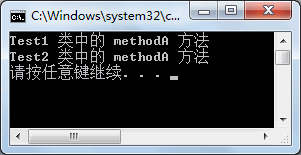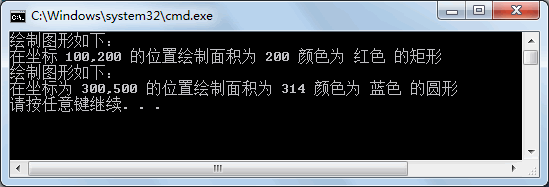# C#接口中多态的实现

< 上一页C#接口的实现 C#集合下一页 >

• 定义接口并使用类实现了接口中的成员。
• 创建接口的实例指向不同的实现类对象。

```interface ITest
{
void methodA();
}
class Test1 : ITest
{
public void methodA()
{
Console.WriteLine("Test1 类中的 methodA 方法");
}
}
class Test2 : ITest
{
public void methodA()
{
Console.WriteLine("Test2 类中的 methodA 方法");
}
}```

```class Program
{
static void Main(string[] args)
{
ITest test1 = new Test1();  //创建接口的实例test1指向实现类Test1的对象
test1.methodA();
ITest test2 = new Test2();  //创建接口的实例test2指向实现类Test2的对象
test2.methodA();
}
}```【实例】创建绘制图形的接口，分别使用两个类来实现接口绘制不同的图形。

```interface IShape
{
double Area { get; }
double X { get; set; }
double Y { get; set; }
string Color { get; set; }
void Draw();
}```

```class Rectangle :IShape
{
//为矩形的长和宽赋值
public Rectangle(double length,double width)
{
this.Length = length;
this.Width = width;
}
public double Length { get; set; }//定义长方形的长度
public double Width { get; set; }//定义长方形的宽度
public double Area
{
get
{
return Length * Width;//计算长方形面积
}
}
public string Color { get; set; }
public double X { get; set; }
public double Y { get; set; }
public void Draw()
{
Console.WriteLine("绘制图形如下：");
Console.WriteLine("在坐标 {0},{1} 的位置绘制面积为 {2} 颜色为 {3} 的矩形", X, Y, Area, Color);
}
}
class Circle : IShape
{
//为圆的半径赋值
{
}
public double Radius { get; set; }
public double Area
{
get
{
}
}
public string Color { get; set; }
public double X { get; set; }
public double Y { get; set; }
public void Draw()
{
Console.WriteLine("绘制图形如下：");
Console.WriteLine("在坐标为 {0},{1} 的位置绘制面积为 {2} 颜色为 {3} 的圆形", X, Y, Area, Color);
}
}```

```class Program
{
static void Main(string[] args)
{
IShape shape1 = new Rectangle(10, 20);
shape1.X = 100;
shape1.Y = 200;
shape1.Color = "红色";
shape1.Draw();
IShape shape2 = new Circle(10);
shape2.X = 300;
shape2.Y = 500;
shape2.Color = "蓝色";
shape2.Draw();
}
}``````Reatangle rectangle = new Rectangle();
rectangle.Length = 10;
rectangle.Width = 20;
IShape shape1 = rectangle;```

< 上一页C#接口的实现 C#集合下一页 >